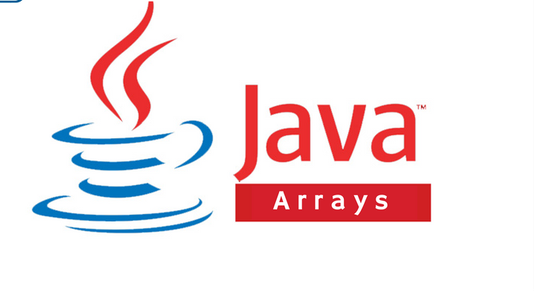# Working With Arrays in JavaArrays refer to a sequential collection of elements of the same type. It is used to store a collection of data. Unlike Javascript where an array can hold many elements of different data types, an array in Java holds a fixed number of values of a single type.

Immediately after creating an array, its length is fixed. Each item in an array is referred to as an element, and each element can be accessed by its numerical index.

An array index begins from 0 and begins to increment for the given length of the array.

Syntax

dataType [] arrayRefValue; //Preferred way

dataTaype arrayRefValue []; //Not so preferred way

Processing Arrays

``````public class TestArray {
public static void main(String [] args) {
double [] myList = {1.2, 1.4, 2.5, 4.5, 1.6}
System.out.println(myList) // 1.2
System.out.println(myList) // 1.4
System.out.println(myList) // 2.5
System.out.println(myList) // 4.5
System.out.println(myList) // 1.6
}
}``````

Summing All Elements in an Array

``````public class TestArray {
public static void main(String [] args) {
double [] myList = {1.2, 1.4, 2.5, 4.5, 1.6}
double total = 0;
for(int i=0; i<myList.length; i++){
total +=myList[i];
}
System.out.println("Total is "+total); //11.2
}
}``````

Finding The Largest Element in an Array

``````public class TestArray {
public static void main(String [] args) {
double [] myList = {1.2, 1.4, 2.5, 4.5, 1.6}
double max = myList;
for(int i=0; i<myList.length; i++){
if(myList[i] > max) max = myList[i];
}
System.out.println("Largest value is "+max); //4.5
}
}``````

See the official Java documentation for Arrays

THE END.